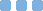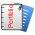Kolkata, , India
Python, Project Management, Electrical Engineering
0 टिप्पणी करें | 0 राय | 31 मई 19  | Pronay Ghosh

The Solar Energy harvesting system has become a significant renewable energy source for different applications. However, I-V characteristic of the solar cell varies with irradiation and temperature and is also nonlinear. We can always see a peak point on the I-V or P-V curve, known as the Maximum Power Point (MPP), on which the operation of the entire photovoltaic cell system depends. At this point, the cell can have the highest efficiency and therefore can generate the maximum output power.
Maximum power point tracking (MPPT) is an algorithm implemented in photovoltaic (PV) inverters to continuously adjust the impedance seen by the solar array to keep the PV system operating at, or close to, the peak power point of the PV panel under varying conditions, like changing solar irradiance, temperature, and load. MPPT algorithms are typically used in the controller designs for PV systems. The mathematical equation developed to define the current-voltage (I–V) relationship of a solar cell is complicated as they have to account for factors such as variable irradiance (sunlight) and temperature to ensure that the PV system generates maximum power at all times. Therefore, the determination of the parameters requires more computational efforts for each operating condition. Previously buck, boost, and buck-boost converters are used to transfer the power generated by PVA to load nowadays an MPPT algorithm directly direct control of duty cycle of the converter. The three most commonly used algorithms are Perturbation and observation (P&O) algorithm, Incremental Conductance algorithm, Fraction Open Circuit Voltage algorithm, Etc.
In this project report, MPPT controller using the P&O algorithm is explored. A generalized Solar cell array model is used with the MPPT Controller (Arduino), and the power outputs of the PV Cell array in various conditions of irradiance and temperature is monitored to verify the effectivity of the algorithm.

• इस ब्लॉग के लिए सामाजिक शेयर

•पोर्फोलिओ और ब्लॉग
Pronay Ghosh विभिन्न कंपनियों का अनुसरण करता है, ये कंपनियां और नियोक्ता Pronay के फिर से शुरू देख सकते हैंसबसे अच्छा नौकरी के अवसर पाने के लिए अपना फिर से शुरू करें अपलोड करें

मुफ्त रजिस्टर करें!